International
Tables for
Crystallography
Volume D
Physical properties of crystals
Edited by A. Authier

International Tables for Crystallography (2006). Vol. D, ch. 1.10, pp. 247-249

## Section 1.10.3. Action of the symmetry group

T. Janssena*

aInstitute for Theoretical Physics, University of Nijmegen, 6524 ED Nijmegen, The Netherlands
Correspondence e-mail: ted@sci.kun.nl

### 1.10.3. Action of the symmetry group

| top | pdf |

#### 1.10.3.1. Action of superspace groups

| top | pdf |

The action of the symmetry group on the periodic density functionin n dimensions is given by (1.10.2.9). The real physical structure, however, lives in physical space. One can derive from the action of the superspace group on the periodic structure its action on the quasiperiodic d-dimensional one. One knows that the density function in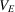is just the restriction of that in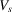. The same holds for the transformed function.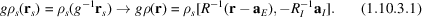This transformation property differs from that under an n-dimensional Euclidean transformation by the `phase shift'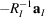. Take for example the IC phase with a sinusoidal modulation. If the positions of the atoms are given by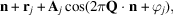then the transformed positions are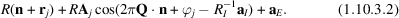If the transformation g is a symmetry operation, this means that the original and the transformed positions are the same.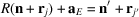and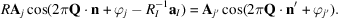This puts, in general, restrictions on the modulation.

Another view of the same transformation property is given by Fourier transforming (1.10.2.9). The result for the Fourier transform is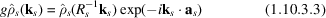and because there is a one-to-one correspondence between the vectors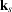in the reciprocal lattice and the vectors k in the Fourier module one can rewrite this as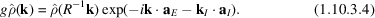For a symmetry element one has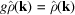. Therefore, the superspace group element g is a symmetry transformation of the quasiperiodic functionif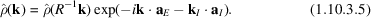This relation is at the basis of the systematic extinctions. If one has an orthogonal transformation R such that this in combination with a translation (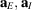) is a symmetry element and such that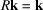, then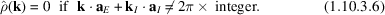Because the structure factor is the Fourier transform of a density function which consists offunctions on the positions of the atoms, for a quasiperiodic crystal it is the Fourier transform of a quasiperiodic function. Therefore, symmetry-determined absence of Fourier components leads to zero intensity of the corresponding diffraction peaks. Therefore, although there is no lattice periodicity for aperiodic crystals, systematic extinctions follow in the same way from the symmetry as in lattice periodic systems if one considers the n-dimensional space group as the symmetry group.

#### 1.10.3.2. Compensating gauge transformations

| top | pdf |

The transformation property of the Fourier transform of the density given in the previous section can be formulated in another way. Consider a functionwhich is invariant under a d-dimensional Euclidean transformation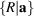in physical space. Then its Fourier transform satisfies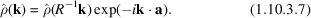Conversely, if the Fourier transform satisfies this relation, the Euclidean transformation is a symmetry operation for. The two equations (1.10.3.5)and (1.10.3.7)are closely related. One can also write (1.10.3.5)as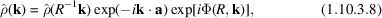where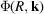can be considered as a gauge transformation that compensates for the phase shift: it is a compensating gauge transformation. It is a function that is linear in k,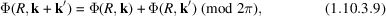and satisfies a relation closely related to the one satisfied by nonprimitive translations.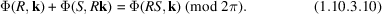[Recall that a system of nonprimitive translationssatisfies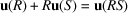modulo lattice translations.] Therefore, the Euclidean transformationcombined with the compensating gauge transformation with gauge functionis a symmetry transformation forif equation (1.10.3.8)is satisfied. This is a three-dimensional formulation of the superspace group symmetry relation (1.10.3.5).

#### 1.10.3.3. Irreducible representations of three-dimensional space groups

| top | pdf |

A third way to describe the symmetry of a quasiperiodic function is by means of irreducible representations of a space group. For the theory of these representations we refer to Chapter 1.2on representations of crystallographic groups.

Consider first a modulated IC phase. Suppose the positions of the atoms are given by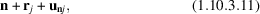where n belongs to the lattice,is a position inside the unit cell and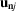is a displacement. If the structure is quasiperiodic with Fourier module, the vectorscan be written as a superposition of normal modes.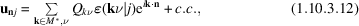where the coefficient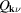is a normal coordinate,denotes the band index and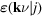denotes the polarization of the normal mode. The normal coordinates transform under a space group according to one of its irreducible representations. The relevant space group here is that of the basic structure. For the simple case of a one-dimensional irreducible representation, for eachthe effect is simply multiplication by a factor of absolute value unity. For example, for the modulated phase with basic space group Pcmn and wavevector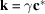there are four non-equivalent one-dimensional representations. It depends on the band index which representation occurs in the decomposition. The space-group element, for which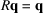(modulo reciprocal lattice) acts onaccording to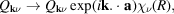where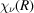is the character of R in an irreducible representation associated with the branch. Because the character of a one-dimensional representation is of absolute value unity, one may write it as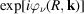. Consequently, if the decomposition of the displacement contains only the vectors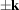, the factordescribes a shift in the modulation function.

Consider again as an example a basic structure with space group Pcmn and a modulation wavevector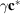. The point groupthat leaves the modulation wavevector invariant is generated byand. This point group mm2 has four elements and four irreducible representations, all one-dimensional. One of them has for the character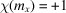,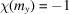. If the displacements of the atoms are described by a normal mode belonging to this irreducible representation, then the compensating phase shifts for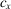andare, respectively, 0 and. In the notation for superspace groups, this is the group Pcmn(00)1s. The same structure can be described by the irreducible representation characterized as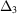, because the modulation wavevector is the pointin the Brillouin zone and the irreducible representationhas the character mentioned above.

In this way there is a correspondence between superspace groups for (3 + 1)-dimensional modulated structures and two-dimensional irreducible representations of three-dimensional space groups.